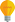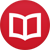# Is 0.999… (repeating) equal to 1?

by Jakub MarianTip: See my list of theMost Common Mistakes in English. It will teach you how to avoid mis­takes with com­mas, pre­pos­i­tions, ir­reg­u­lar verbs, and much more.

The short answer is yes. $0.\bar 9$ (zero point 9 repeating) is exactly 1. However, a lot of people find this result counter-intuitive (people often feel that $0.\bar 9$ should be slightly less than 1), but this feeling stems from a misunderstanding of what $0.\bar 9$ means.

To satiate those looking for a quick and dirty argument, we can do the following simple calculation:

\begin{align*} x &= 0.999..{}.\\ 10x &= 9.999..{}.\\ 9x &= 10x - x = 9.999..{}.-0.999..{}. = 9\\ x &= 1 \end{align*}

This is not, however, a mathematical proof, because not all of the steps are obvious without further looking at the definition of the decimal notation. This will require a bit of preparation.

## The limit

You are probably familiar with the meaning of the decimal notation when the fractional part contains only a finite number of digits. For example:

$$32.58 = 3⋅10+2⋅1+5⋅÷{1}{10}+8⋅÷{1}{100}$$

To understand the meaning of the fractional part when the digits go on indefinitely, you first need to understand, at least purely intuitively, the notion of limit.

When you have a sequence of numbers, say $a_1 = 1$, $a_2 = ÷{1}{2}$, $a_3 = ÷{1}{3}$, …, $a_n = ÷{1}{n}$, you can compute what we call the limit of $a_n$ (at $∞$). The name sounds terrifying, but the concept is in fact really simple.

As you can see (or quickly check using a calculator), $÷{1}{n}$ gets closer and closer to 0 as $n$ increases, for example, $÷{1}{100} = 0.01$, $÷{1}{1000} = 0.001$, and so on. If a sequence gets arbitrarily close to a number, we say that the limit of the sequence is that number, and we write:

$$\lim_{n→∞} a_n = \text{the number to which a_n get arbitrarily close}$$

For example, in the case of $a_n = ÷{1}{n}$, we would write

$$\lim_{n→∞} a_n = 0$$ since $÷{1}{n}$ gets arbitrarily close to $0$ for large $n$. Now we are ready to define the decimal notation for never-ending sequences of digits.

## Real numbers and the decimal notation

It may come as a great surprise to those who don’t know any higher mathematics, but the real numbers are not defined to be “numbers like $0.53$, $3.1415$…, $86.51$”. In fact, we define them in three steps:

1) Define the integers (whole numbers), i.e. numbers like $1$, $5$, $-8$.
2) Define fractions of integers, i.e. numbers like $÷{5}{8}$, $÷{314}{100}$.
3) Add abstract numbers that fill in “gaps” between fractions.

The mathematical definition is somewhat more technical, but it follows the process outlined above. There is absolutely no mention of the decimal point anywhere in the process; we just have a bunch of numbers, such as $1$, $÷{3}{7}$, or $π$, and then, after we have defined the numbers, we define the decimal point.

This is simple if the decimal part is finite (we already did this above), but what if it is infinite? We use the limit! For example, to get the value of $x = 0.999$…, we define a sequence of the form $x_1 = 0.9$, $x_2 = 0.99$, $x_3 = 0.999$, and so on. Obviously, as $n$ gets larger, $x_n$ gets closer to the real value of $0.999$…, so we define:

$$0.\bar 9 = \lim_{n→∞} x_n$$

The only question that remains to be answered is: What is the limit of $x_n$? As $n$ gets larger, $x_n$ gets arbitrarily close to $1$, so we conclude that, by definition:

$$0.\bar 9 = \lim_{n→∞} x_n = 1$$

The phrase “by definition” is really important here. Symbols like “1.234” are not real numbers themselves; these are just just a way to label real numbers, just like we label humans with words like “Peter” or “Laura”it wouldn’t really make sense to say that a person is a series of letters, would it… And just like “Peter” may be also called “Pete”, some numbers can be written in two different ways in our notation: $0.999$… is just a different way of writing $1$.

By the way, I have written several educational ebooks. If you get a copy, you can learn new things and support this website at the same time—why don’t you check them out?